Question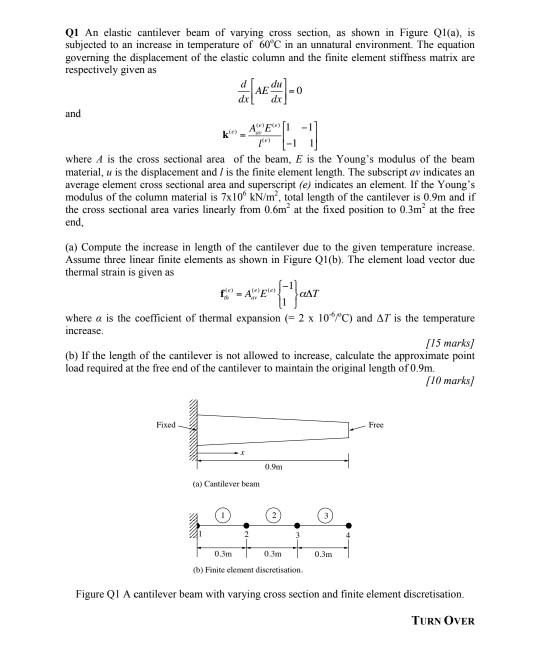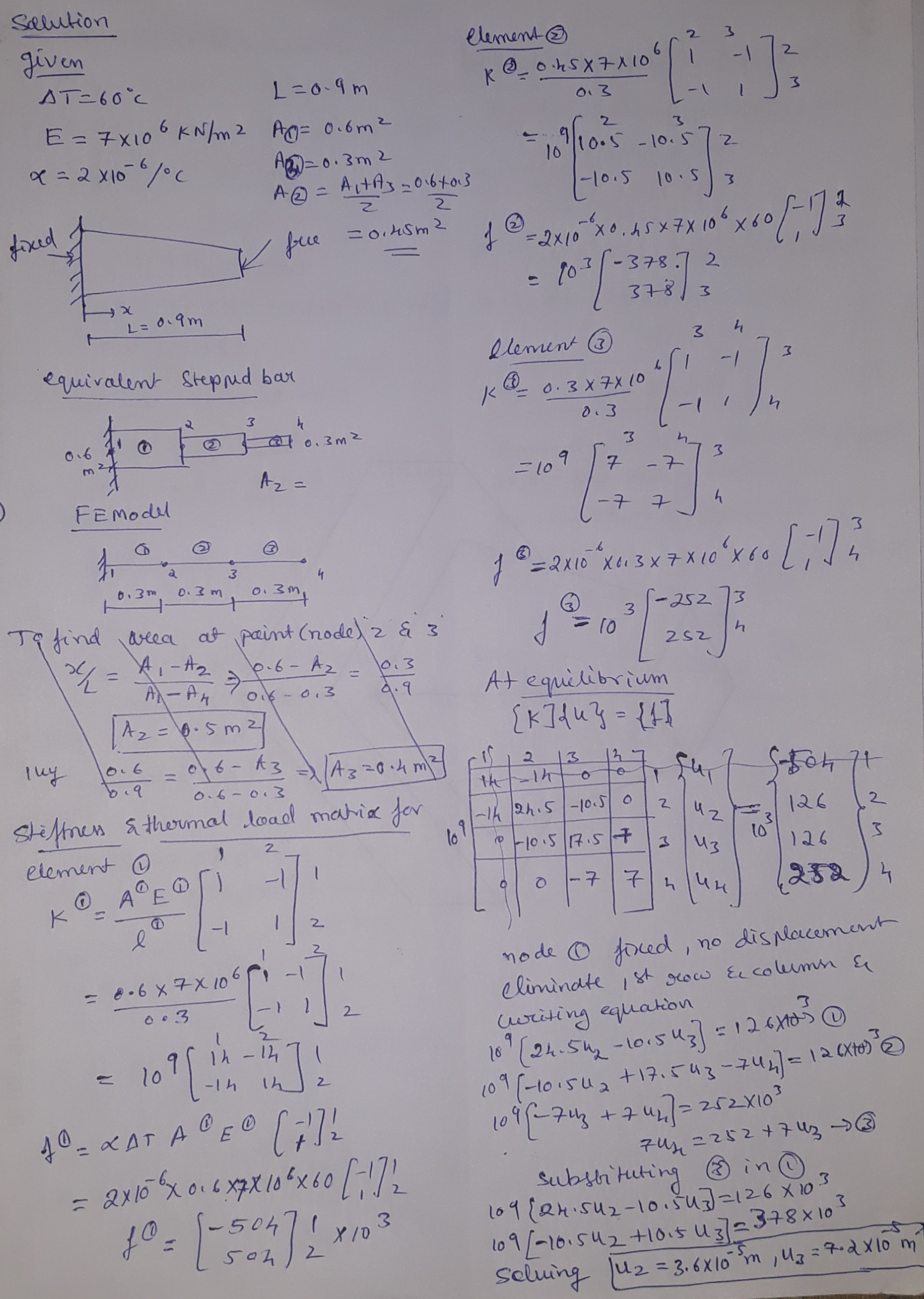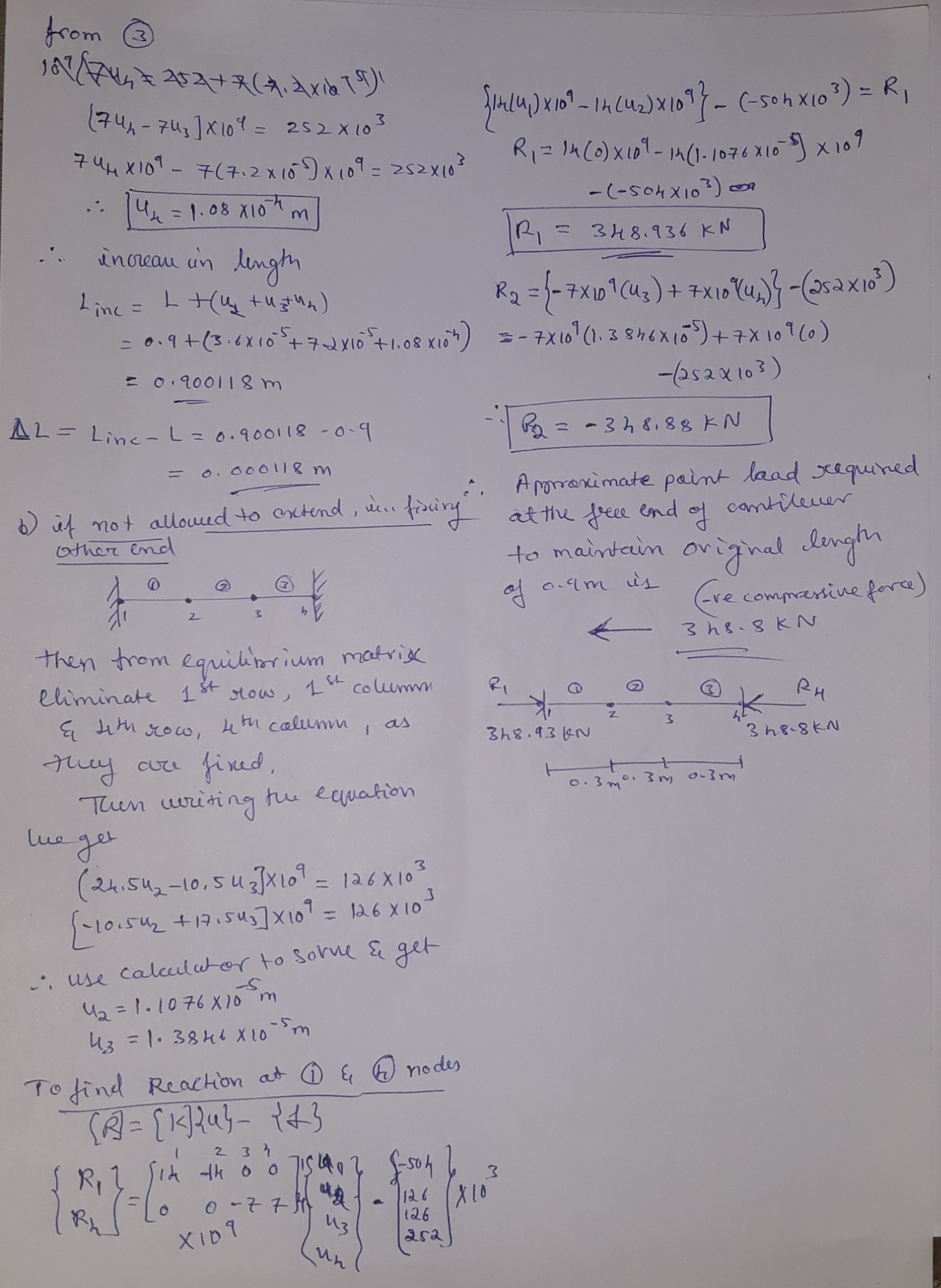#### Earn Coins

Coins can be redeemed for fabulous gifts.

Similar Homework Help Questions
• ### need to solve the mathematical model to prove that we can get the equations i Q1...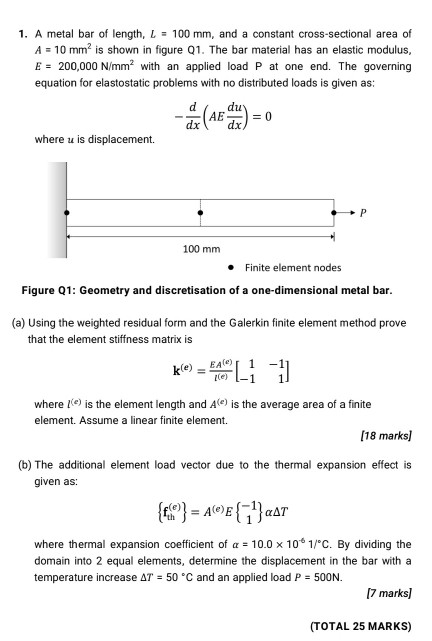need to solve the mathematical model to prove that we can get the equations i Q1 a methematically please use only the weighted resedual and gerkins methods to prove it 1. A metal bar of length, L = 100 mm, and a constant cross-sectional area of A = 10 mm? is shown in figure Q1. The bar material has an elastic modulus, E = 200,000 N/mm2 with an applied load P at one end. The governing equation for elastostatic problems...

• ### 8. The cantilever beam in Figure Q8 subjects to concentrated loading. The cross section geometry gives...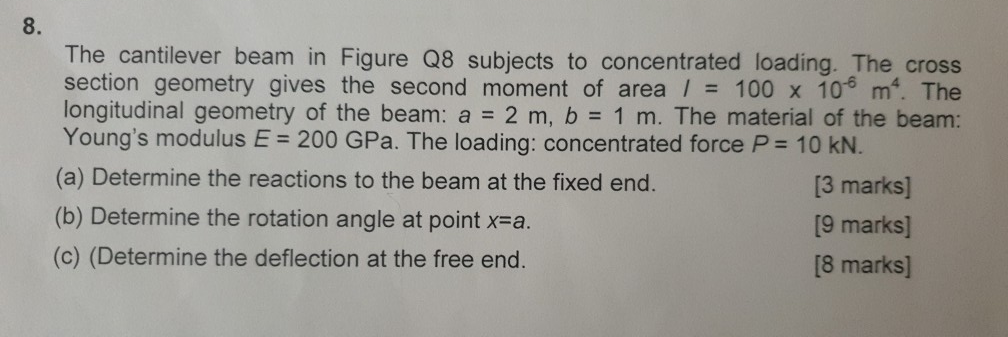8. The cantilever beam in Figure Q8 subjects to concentrated loading. The cross section geometry gives the second moment of area / 100 x 10 m. The longitudinal geometry of the beam: a 2 m, b 1 m. The material of the beam: Young's modulus E 200 GPa. The loading: concentrated force P 10 KN. (a) Determine the reactions to the beam at the fixed end. (b) Determine the rotation angle at point x-a (c) (Determine the deflection at the...

• ### A cantilever beam with a Z-shaped cross section is subjected to a force at the tip...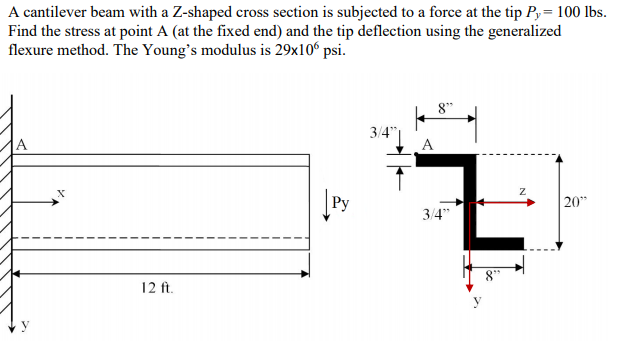A cantilever beam with a Z-shaped cross section is subjected to a force at the tip Py = 100 lbs. Find the stress at point A (at the fixed end) and the tip deflection using the generalized flexure method. The Young's modulus is 29x100 psi. 20" - - - - - - - - - - - - - - -- 12 ft.

• ### A cantilever beam of a channel section is loaded at its half-length, as shown in Figure Q2. The Young's modulus of the material is 200 GPa. Determine the deflection at the free end. [12.5 marks]...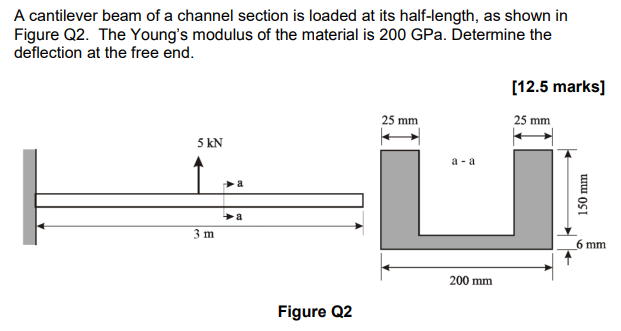A cantilever beam of a channel section is loaded at its half-length, as shown in Figure Q2. The Young's modulus of the material is 200 GPa. Determine the deflection at the free end. [12.5 marks] 25 mm 25 mm 5 kN a -a 少a 6 mm 200 mm Figure Q2 A cantilever beam of a channel section is loaded at its half-length, as shown in Figure Q2. The Young's modulus of the material is 200 GPa. Determine the deflection at...

• ### A cantilever beam AB with a circular cross section and length L = 750 mm supports...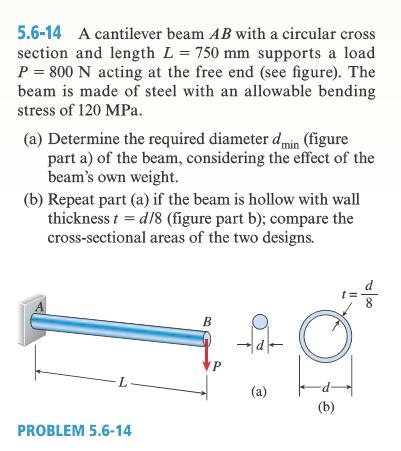A cantilever beam AB with a circular cross section and length L = 750 mm supports a load P = 800 N acting at the free end (see figure). The beam is made of steel with an allowable bending stress of l20 MPa. Determine the required diameter d_min (figure part a) of the beam, considering the effect of the beam's own weight. Repeat part (a) if the beam is hollow with wall thickness t = d/8 (figure part b); compare...

• ### Carry out analysis of a beam hanging under its own weight in a 1d space using the finite element ...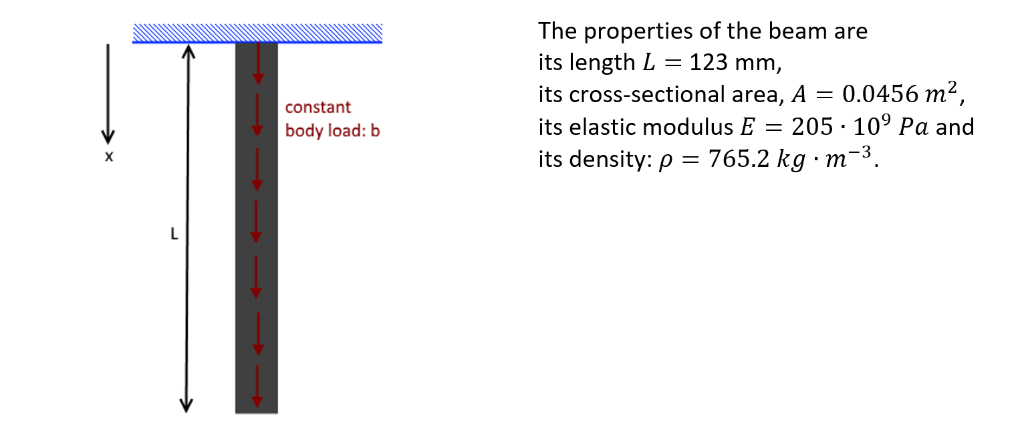Carry out analysis of a beam hanging under its own weight in a 1d space using the finite element 10-step procedure. Carry out the analysis using a single 1d quadratic element. The figure below contains the properties for the beam. The properties of the beam are its length L123 mm, its cross-sectional area, A 0.0456 m2 its elastic modulus E205 -109 Pa and its density: p 765.2 kg m-3 constant body load: b The properties of the beam are its...

• ### need to solve the mathematical model to prove that we can get the equations i   Q1...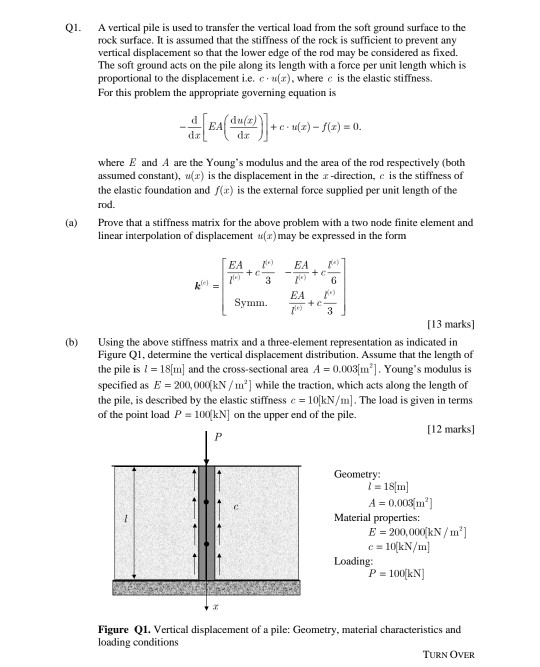need to solve the mathematical model to prove that we can get the equations i   Q1 a methematically QI. A vertical pile is used to transfer the vertical load from the soft ground surface to the rock surface. It is assumed that the stiffness of the rock is sufficient to prevent any vertical displacement so that the lower edge of the rod may be considered as fixed. The soft ground acts on the pile along its length with a force...

• ### Q5. The cantilever beam, AC, is subjected to the load case shown in Figure 5. For the loading shown, do the following: [10 Marks] a) Calculate the magnitude and direction of the reactions at A b) Usi...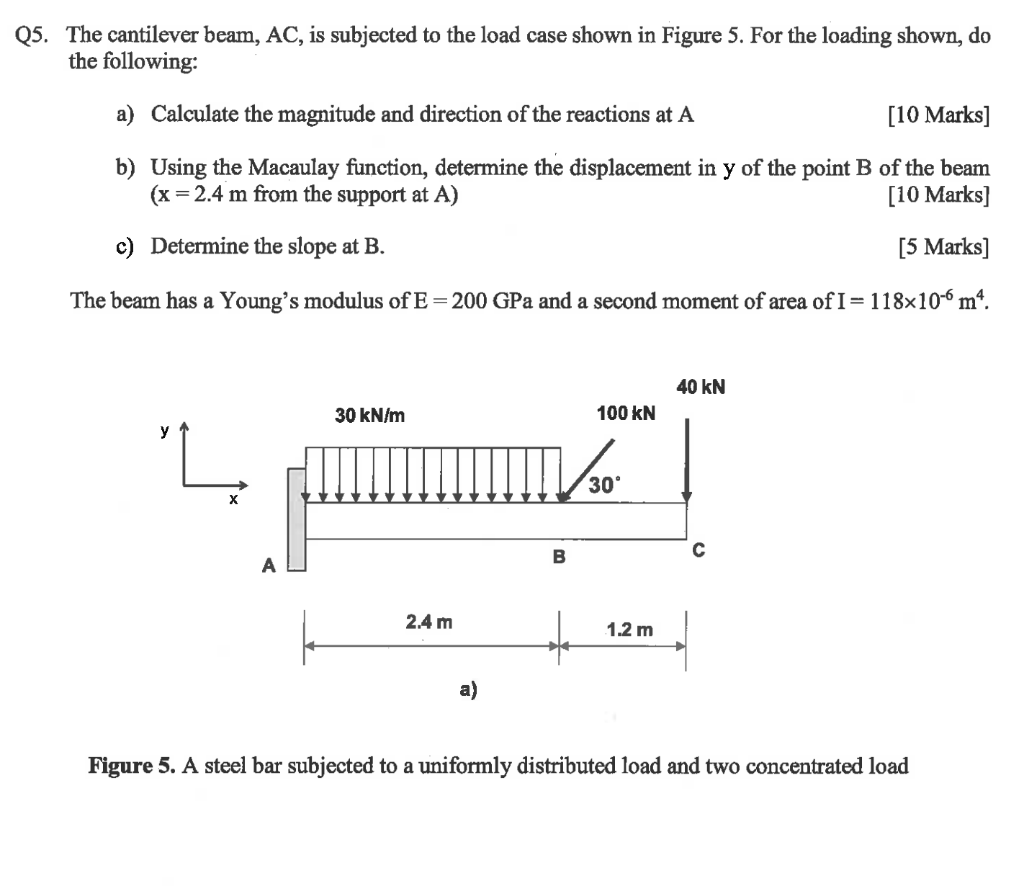Q5. The cantilever beam, AC, is subjected to the load case shown in Figure 5. For the loading shown, do the following: [10 Marks] a) Calculate the magnitude and direction of the reactions at A b) Using the Macaulay function, determine the displacement in y of the point B of the beam (x 2.4 m from the support at A) [10 Marks] c) Determine the slope at B. [5 Marks] The beam has a Young's modulus of E-200 GPa and...

• ### Problem 1 A composité beam made of concrete (c) and six steel Steel bars (s) bars...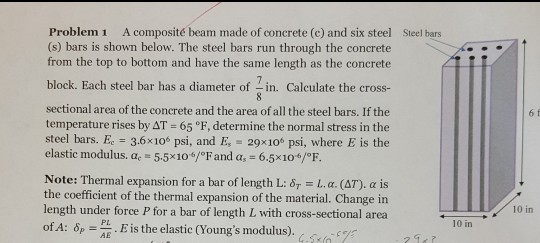Problem 1 A composité beam made of concrete (c) and six steel Steel bars (s) bars is shown below. The steel bars run through the concrete from the top to bottom and have the same length as the concrete block. Each steel bar has a diameter of in. Calculate the cross- sectional area of the concrete and the area of all the steel bars. If the temperature rises by AT 65 °F, determine the normal stress in the steel bars....

• ### A truncated cone with a circular cross-section is suspended as shown and subjected to a force...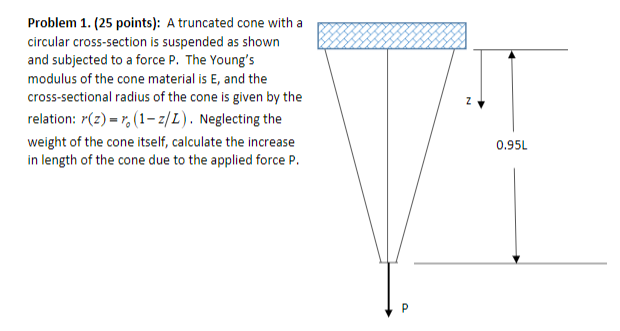A truncated cone with a circular cross-section is suspended as shown and subjected to a force P. The Young’s modulus of the cone material is E, and the cross-sectional radius of the cone is given by the relation: rz r zL () 1 = ? o ( ) . Neglecting the weight of the cone itself, calculate the increase in length of the cone due to the applied force P. Problem 1. (25 points): A truncated cone with a8886 circular...